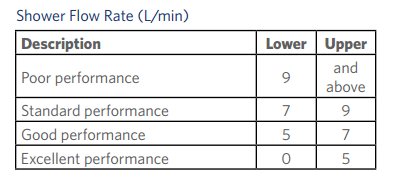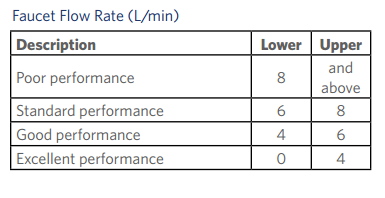Sefaira currently doesn't take into account Domestic Hot Water load and this is something that you may want to calculate as part of your performance analysis.

To help you calculate this we've developed a spreadsheet that does exactly that. It is quite simple but should give you a good approximation of your DHW load.

Simply download the spreadsheet and go to sheet called "input". You first need to input the monthly ground temperature. We use this to estimate the incoming water temperature and you can find these values on the internet. Then input how many people are in the building and how many water fixtures you have.

In the Output tab you will find your total and monthly DHW load.

We can easily edit this spreadsheet so let us know if you wish us to add more input/output . Also, let us know if this is something you would like to see within Sefaira.

You can find below typical flow rate values:•